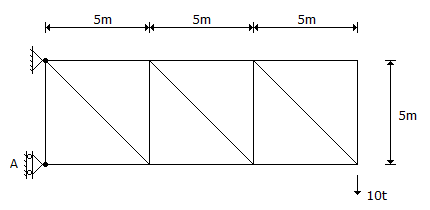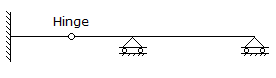# Civil Engineering - UPSC Civil Service Exam Questions

31.

Which one of the following statements is correct ?
In a plane truss, influence line values

 A. vary parabolically between panel points B. vary exponentially between panel points C. vary linearly between panel points D. vary following a cubic relationship between panel points

Explanation:

No answer description available for this question. Let us discuss.

32.

In a 4 m wide rectangular channel with 0.8 m depth of flow, a sharp-crested weir of 2.5 m length with its sill 0.3 m above the channel bed is fixed symmetrically across the width of the channel. Taking the flow to be free but with end contractions, what would be the discharge neglecting approach velocity effect? (Take Cd = 0.625)

 A. 1.39 m3/s B. 1.42 m3/s C. 1.49 m3/s D. 1.56 m3/s

Explanation:

No answer description available for this question. Let us discuss.

33.

A structural member carrying a pull of 700 kN is connected to a gusset plate using rivets. If the pulls required to shear the rivet, to crush the rivet and to tear the plate per pitch length are respectively 60 kN, 35 kN and 70 kN, then the number of rivets required will be

 A. 22 B. 20 C. 18 D. 12

Explanation:

No answer description available for this question. Let us discuss.

34.

In the cantilever truss shown in the given figure, the reaction at A isA. 10 t B. 20 t C. 30 t D. 40 t

Explanation:

No answer description available for this question. Let us discuss.

35.

The degree of indeterminacy of the beam given in the given figure isA. zero B. one C. two D. three